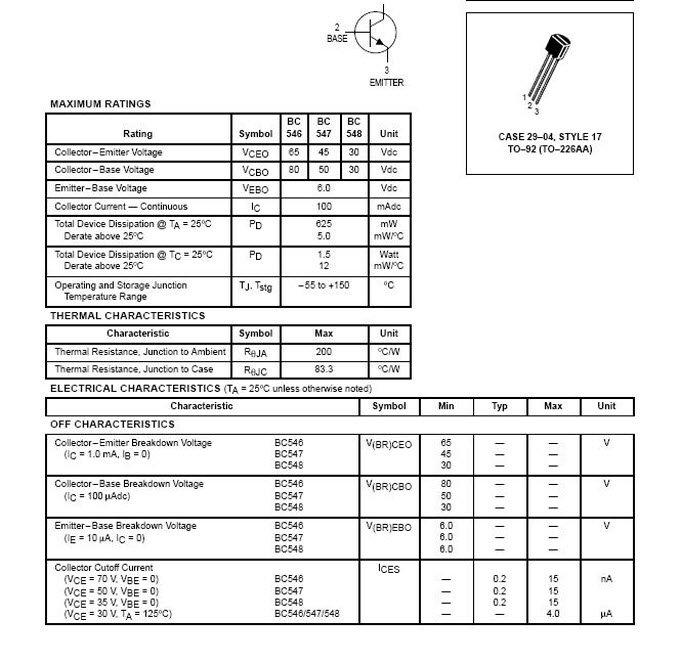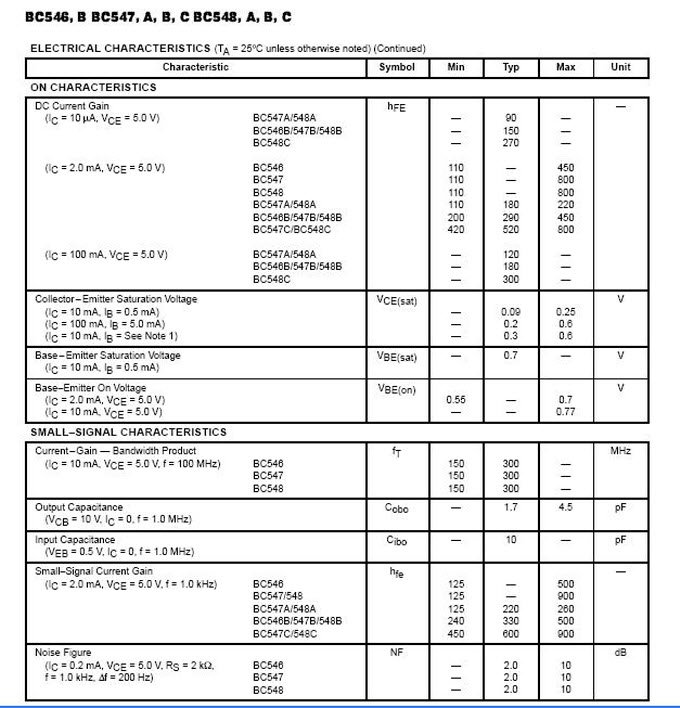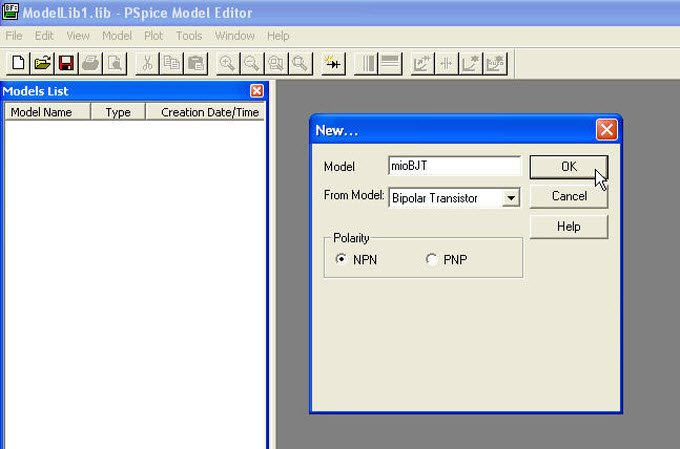# SPICE modeling of a BJT from Datasheet

All these parameters are used by SPICE to represent the different functions that govern behavior of the device in different situations. For example, base current in DC is calculated as:

Ib = Junction surface *(Ibe1/BF + Ibe2 + Ibc1/BR + Ibc2),

where BF e BR are known parameters,

Ibe1 = Direct Diffusion Current = IS*(e^(Vbe/(NF*Vt)) – 1)

Ibe2 = Base Emitter Current = ISE*(e^(Vbe/(NE*Vt)) – 1)

Ibc1 = Inverse Diffusion Current = IS*(e^(Vbe/(NR*Vt)) – 1)

Ibc2 = Base Collector Current = ISC*(e^(Vbe/(NC*Vt)) – 1)

Similarly there are functions for the collector current , for the determination of the base-emitter capacitance, base-collector capacitance, and features that investigate the influence of temperature for different parameters.

In this case we’ ll model a BJT transistor, NPN type, the BC548A, from datasheet info. Unlike the diode in which we could obtain different data directly from datasheet and from semi-empirical rules, in this case we have few data that can be used directly, most of the parameters have to be extracted from datasheet graphs through the use of PSpice Model Editor tools.

We begin with the parameters that can be set to their default values without significant influence on the modeling. let’s Assume XTI=3, EG=1.11 eV, XTB=0, TR=10ns, FC= 0.5. See below first pages of the datasheet for bc548a:The only information we can use directly is essentially the maximum value of fT at 300Mhz, which allows us to estimate TF= 1/(6.28* 300 * 10^6)= 530 ps.Let’s see other parameters obtained from the graphs of the datasheet.Open PSpice Model Editor and give a name to our model, choosing the type of BJT:Posted in PSpice Modeling from Datasheet and tagged , , , .